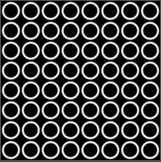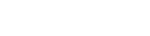Shaders are programs written in Shader Language that run very fast on the GPU (Graphics Processor) of your Intel CPU or on the dedicated NVIDIA/AMD/MSI Graphics Card. They accept input data and affect the individual pixels of an image in terms of their position on the screen and their mapping in relation to the UV texture (2D image) that is associated with it.

In the code, there are Uniforms to input parameters, Varyings for global variables, a Vertex function to handle geometry, a Fragment function to affect Colors, and a Lighting function.

A key thing to bear in mind is that shaders do not store data between operations at the various positions of the vertex points and UV coordinates. So you can’t set a variable that is used between points like you can do in GDScript.

## Materials

In Godot, any Node that inherits from a Canvas Item will have a Material slot in the Inspector.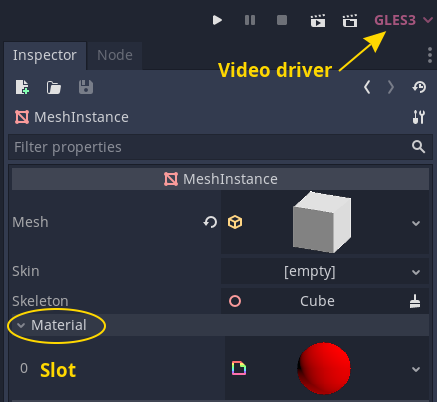We may create a New Shader Material which itself has a Shader parameter. For this we may edit the code for the Shader or create it in the Visual Editor.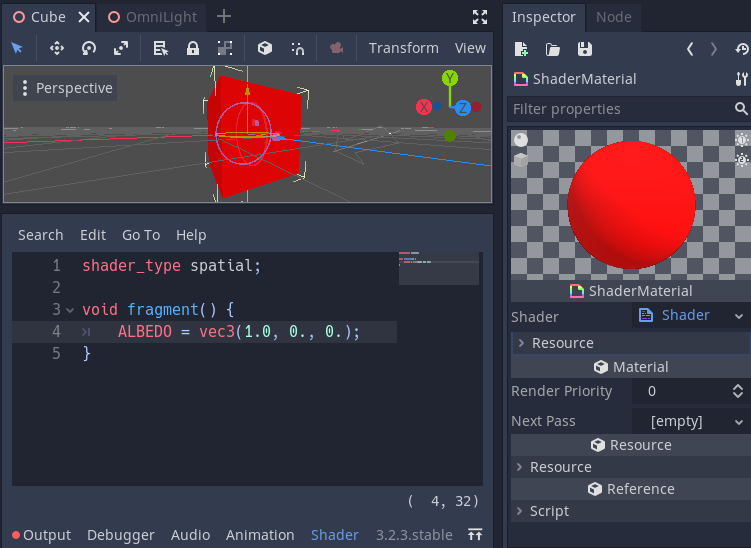## Input Data

Input data are called Uniforms. This data may be simple numbers, color values, textures, or matrices. The Godot Editor allows us to decorate the input parameters such that the user interface presents us with a nice way to enter the relevant data.

## Vertex Coordinates

In 2D, an image is a rectangle (Sprite or Texture Rectangle) that has 4 corners (4 vertices). The coordinate values are based on the size in pixels of the image texture. The middle of the image has a coordinate of 0,0 even though there is no vertex point there. Imaginary points between the vertices are interpolated and may be used in operations in the Fragment Shader.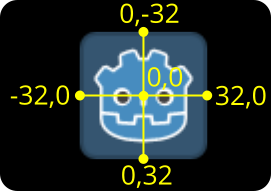In 3D, a mesh defines the shape of the 3D object with any number of vertices with x,y,z coordinates based on pixels.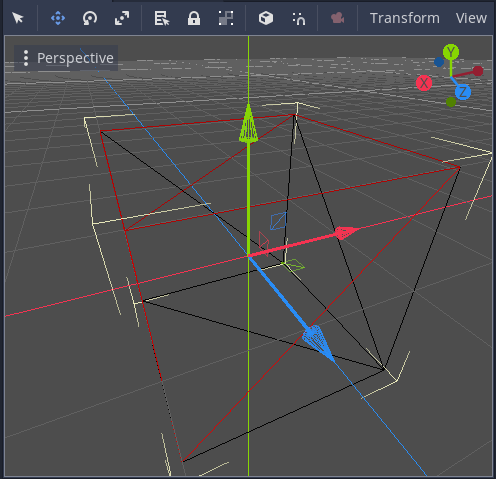## UV Coordinates

In 2D we use images and in 3D we have textures which are 2D image files defining the color, depth, surface normals etc. The coordinates of points on these images use floating point numbers ranging from 0 to 1.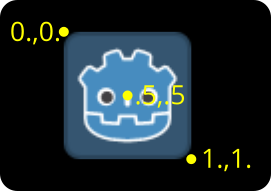Textures are wrapped around the surfaces of a 3D object according to the UV mapping data stored in the object model.

Here is a UV suitable for mapping faces onto a cube. Notice how some areas are wasted and parts of the image are distorted so that it may fit into a square image shape.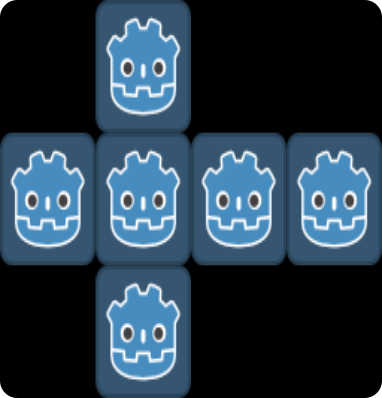As per the 2D image, coordinates ranging from 0,0 to 1,1 map the points of the image to areas on the cube surfaces.

With a shader, we might input the UV as a texture uniform. But we may also generate the colors with code based on the UV coordinate, vertex coordinate, and time.

In code it is very convenient to deal with floating point values ranging from zero to one because we can simply take the fractional part of a number as a valid UV value, and also use absolute values of sine and cosine functions. Also, vectors may be normalized to fit in the UV range.

## 2D Transformations

By changing the Vertex point values we may scale, shear, and rotate a 2D image. Each point is passed to the Vertex shader as a 2D vector. The shading language is not GDScript but more like C. Let’s show some example scripts.

To set up an experimental scene you can simply add a sprite to a Node2D. Then create a new shader material in the Material slot of the inspector panel. Then edit a new shader script.

Start by adding `shader_type canvas_item;` to specify the type of shader that we will create.

Then we will add our code to the vertex shader function. Various data values are available called “built-ins”, we are interested in the VERTEX value. This is a vec2 which contains the points of a vertex point pixel position on the canvas of the image.

Bear in mind that a sprite’s geometry is made up of 2 triangles and hence, only has 4 vertices that may be moved. So this limits us as to how much we can bend and twist its shape.

### Scale

``````shader_type canvas2D;

void vertex() { // The vertex shader function
VERTEX *= 2.; // Transform the point coordinates
}
``````

Here we simply double the point coordinate values so that the image is scaled by x2. We used shorthand code for the multiplication rather than writing `VERTEX = VERTEX * 2.0`.

We must include a decimal point for the number to indicate that it is a floating point value, otherwise it will be interpreted as an integer. Also, superfluous zeros don’t need to be included in shader code.

### Shear

Shear is the same as tilting the edges of the image. To do this we want to shift the x coordinates sideways by a linear amount proportional to y. Hence, add a y displacement to x.

``````shader_type canvas2D;

void vertex() {
VERTEX.x += VERTEX.y;
}
``````

Here we use dot notation to access the x and y values of the vector and use a shorthand addition.

### Inputs

To dynamically modify the vertex points we may make use of the built-in TIME value which is constantly changing or provide uniforms. Uniforms are input values that are accessible in the inspector or via our game scripts.

We may take the fractional part of the time value to give us a ramp from 0 to 1 repeating every second. To vary the rate, we may multiply TIME by a factor. Now we may apply this ramp to a sine function to get a sine wave swinging between -1 and +1. Then scale and shift this to keep it within a range of say 0.5 to 1.0.

This can be used to create a pulsing effect of the sprite as follows:

``````shader_type canvas2D;

void vertex() {
float pi = 3.141592653589793;
VERTEX *= .5 + (sin(fract(TIME) * pi * 2.) + 1.) / 4.;
}
``````

To add a rate input we may add a uniform as follows:

``````shader_type canvas2D;

uniform float rate = 1.0;

void vertex() {
float pi = 3.141592653589793;
VERTEX *= .5 + (sin(fract(TIME * rate) * pi * 2.) + 1.) / 4.;
}
``````

Now this rate value will appear as a shader parameter in the inspector, and may be changed to alter the rate of the pulsating effect. To change the value in GDScript we may do this:

``````get_node("Sprite").get_material().set_shader_param("rate", 2.2)
``````

### Rotation

Rotation makes use of a transformation matrix. This is fed with the angle of rotation and the vertex points are transformed by the matrix.

``````shader_type canvas_item;

uniform float rate = 1.0;

void vertex() {
float pi = 3.141592653589793;
float a = fract(TIME * rate) * pi * 2.; // Rotate over full circle
VERTEX = mat2(vec2(cos(a), sin(a)), vec2(-sin(a), cos(a))) * VERTEX;
}
``````

A 2X2 matrix may be constructed from a couple of vec2 vectors. Note that we didn’t use the short form for multiplication here because with matrix multiplication, the evaluation order is important to get the expected result.

There are also standard linear transformation matrices for shear, scale, and reflection etc.

Using this shader, we are able to affect the appearance of the pixels of the image. We may modify the input texture or replace it entirely with generated values.

Some useful built-in values:

• UV - the position of the current pixel
• COLOR - the color of the current pixel
• TEXTURE - the sprite’s image

Let’s explore some examples.

### Convert color image to monochrome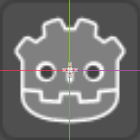This can be achieved by averaging the RGB color values to give equal output values for the COLOR.rgb vector. But to take into account human perception of color we may apply a weighted average.

``````shader_type canvas_item;

void fragment() {
COLOR = texture(TEXTURE, UV); // Get pixel color from the image texture
float grey = .21 * COLOR.r + .71 * COLOR.g + .07 * COLOR.b;
COLOR.rgb = vec3(grey); // Change the color value
}
``````

Note that above we are able to set all 3 components of a vec3 with one float input value.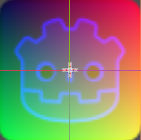``````shader_type canvas_item;

void fragment() {
COLOR = texture(TEXTURE, UV); // Get the sprite pixel rgb color
COLOR.rg = UV.xy; // Map UV x,y values to COLOR red, green values
}
``````

### Passing values between the vertex and fragment shader

In this example, we will make use of the convenience that the vertex origin is in the center of the sprite and pass its value from the vertex shader to the fragment shader using a varying. Then we can evaluate the length of the vector and apply our effect or not.

``````shader_type canvas_item;

uniform float rate = 1.0;

varying vec2 v; // This is our varying value yet to be assigned

void vertex() { // Do some spinning
float pi = 3.141592653589793;
float a = fract(TIME * rate) * pi * 2.;
VERTEX = mat2(vec2(cos(a), sin(a)), vec2(-sin(a), cos(a))) * VERTEX;
v = VERTEX; // Here we assign the value to our varying
}

void fragment() {
COLOR = texture(TEXTURE, UV);
if (length(v) > 16.) // Do our shady stuff outside of a radius of 16px
COLOR.rg = UV.xy;
}
``````

In the above code, you can see how we may affect only certain areas of the image by using a conditional statement.

Due to the extra dimension of depth along the z axis, there is more to consider when working with 3D shaders. For example, some faces of the object may be obscured from view. Also, the UV coordinates relate texture pixels to positions on the faces depending on a map.

In Godot, a Spatial node such as a MeshInstance has a mesh property that defines all the vertex points making up the wire frame of it. Then it is wrapped by a material. This material has many properties including Albedo (color or texture), Normal map, and Transmission.

Hopefully, I will post more on the big topic of shaders later. And this page serves as a useful introduction to Shaders in Godot Engine.

## Drawing Lines

The UV value may be used as the current point in the texture area. So to draw a line, we need to display some color when the UV is very close to or on a point of our line, and not display color elsewhere.

Let’s draw a vertical line around one third of the way along the x-axis. This corresponds to `UV.x == 0.3`.

We can calcuate the distance that the UV is away from the line with: `abs(UV.x - x)`

Using the smoothstep function we can interpolate between 2 values where the first is for dark and the second for bright. This small difference in values will correspond to half the thickness of our line which is related to how far away from the line position the UV is at.

The values are defining where the start and end edges of the step output from 0.0 to 1.0 occur in response to the 3rd parameter input. The 3rd parameter value traverses this range as the UV passes over our line position. The smoothing softens the edges of the line.

So we may write a function to draw lines like so:

``````float line(vec2 pos, float x) {
return smoothstep(0.01, 0.0, abs(pos.x - x));
}
``````

`x` is where we want the line to appear, and `pos.x` is the current position of the fragment shader.

And the shader code may look like:

``````shader_type canvas_item;

float line(vec2 pos, float x) {
return smoothstep(0.01, 0.0, abs(pos.x - x));
}

void fragment() {
COLOR.rgb = vec3(line(UV, 0.3));
}
``````

## Drawing Circles

For a line drawn in a circle, we can compare the UV value to the radius and offset of the circle.

It’s easier if we take out the offsets. For the UV which ranges from `0,0` to `1,1` we may transform this to originate in the center. `vec2 uv = UV - 0.5;`

So we may write a shader to draw circles like so:

``````shader_type canvas_item;

float circle(vec2 pos, float radius) {
}

void fragment() {
COLOR.rgb = vec3(circle(UV - 0.5, 0.4));
}
``````

## Repeating Patterns

In the above examples, we match the value of a UV coordinate with a position. To match the value multiple times to say create a grid of patterns, we may multiply UV by a factor and take the fractional part as the UV value.

To create a grid of circles:

``````shader_type canvas_item;

float circle(vec2 pos, float radius) {